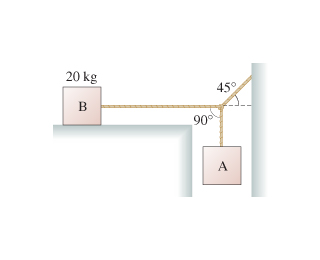# Problem: Block B in the figure(Figure 1) rests on a surface for which the static and kinetic coefficients of friction are 0.63 and 0.40, respectively. The ropes are massless.What is the maximum mass of block A for which the system remains in static equilibrium?Express your answer to two significant figures and include the appropriate units.

###### FREE Expert Solution

Static frictional force by B = T

T = μsmg

The force balance weight of block A.###### Problem Details

Block B in the figure(Figure 1) rests on a surface for which the static and kinetic coefficients of friction are 0.63 and 0.40, respectively. The ropes are massless.What is the maximum mass of block A for which the system remains in static equilibrium?

Express your answer to two significant figures and include the appropriate units.